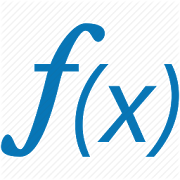## Functions and Relation - Lumos Educational App Store41
Price -fREE
\$0

#### DESCRIPTION:

This app contains basic material of function and Relation.In mathematics, a function is a relation between a set of inputs and a set of permissible outputs with the property that each input is related to exactly one output. An example is the function that relates each real number x to its square x2. The output of a function f corresponding to an input x is denoted by f(x) (read "f of x"). Following parts of function are discussed in the app 1) Cartesian Product of sets 2) Relation 3) Types of function 4) Surjective function 5) Injective function 6) Bijective function 7) Composition of

#### OVERVIEW:

Functions and Relation is a free educational mobile app By .It helps students in grades HS practice the following standards HSF.IF.A.1.

This page not only allows students and teachers download Functions and Relation but also find engaging Sample Questions, Videos, Pins, Worksheets, Books related to the following topics.

1. HSF.IF.A.1 : Understand that a function from one set (called the domain) to another set (called the range) assigns to each element of the domain exactly one element of the range. If f is a function and x is an element of its domain, then f(x) denotes the output of fcorresponding to the input x. The graph of f is the graph of the equation y = f(x)..

HS

#### STANDARDS:

HSF.IF.A.1

Developer:

Software Version: 5

Category:

### RELATED APPSEdSearch WebSearch